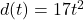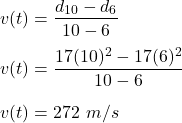## An object dropped from rest from the top of a tall building on Planet X falls a distance d (t )equals 17 t squared feet in the first t secon

Question

An object dropped from rest from the top of a tall building on Planet X falls a distance d (t )equals 17 t squared feet in the first t seconds. Find the average rate of change of distance with respect to time as t changes from t1= 6 to t2=10. This rate is known as the average​ velocity, or speed.

in progress 0
6 months 2021-07-15T02:40:34+00:00 1 Answers 41 views 0

## Answers ( )

The average speed of the object is 272 m/s.

Explanation:

Given that,

An object dropped from rest from the top of a tall building on Planet X falls a distance of :t is time in seconds

We need to find the average rate of change of distance with respect to time as t changes from t = 6 s to t =10 s. The rate of change of distance wrt time is called velocity of an object. Average velocity is given by :So, the average speed of the object is 272 m/s.